# College Chemistry : Half-Reactions and Redox Potentials

## Example Questions

### Example Question #4 : Redox Reactions

Consider the following redox reaction.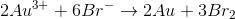If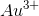has a reduction potential of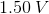and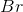has a reduction potential of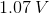, what is the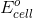for this redox reaction?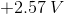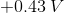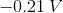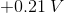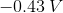Explanation:

In this question, we're given an overall redox reaction as well as the relevant reduction potentials, and we're asked to solve for.

To begin with, we can show each of the individual half reactions to make it more clear.

For gold: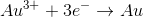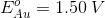For bromine: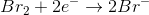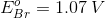Note that the reduction potential for each of these half reactions is positive. This means that for both elements, their reduction is spontaneous. The more positive the voltage (or the less negative), the more spontaneous it is.

From the overall question given to us in the question stem, we see that bromine is not being reduced but, rather, it is being oxidized. Since oxidation is the reverse of reduction, the reduction potential maintains the same magnitude but the sign in front of it changes. Thus, the oxidation of bromine has a value of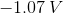. Furthermore, because the overall reaction shows gold being reduced, we don't need to change the sign of gold's reduction potential.

To find the overallfor the reaction, we simply just add these two values together.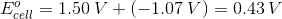One very important thing to note is that we did not need to multiply the reduction potential for either of the half reactions. Even though the reduction reaction for gold needs two stoichiometric equivalents, and bromine's oxidation needs three stoichiometric equivalents, the values of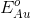and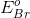do not change. This is because the any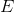value represents an intrinsic property. In other words, the value is not dependent on the amount of material present. As you add more material, it is true that there will be greater electron flow. But at the same time, there will also be more energy change as these electrons flow. The consequence is that both of these values (electron flow and change in energy) change proportionately, such that their ratio will always equal the value ofthat is characteristic of that redox reaction.

### Example Question #5 : Redox Reactions

The standard reduction potential of silver is equal to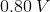. If silver acts as the anode in a voltaic cell, which of the following would be able to act as the cathode?

Nickel, with a standard reduction potential of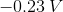Sodium, with a standard reduction potential of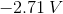Cobalt, with a standard reduction potential equal to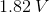Copper, with a standard reduction potential equal to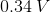Cobalt, with a standard reduction potential equal toExplanation:

In this question, we're given the standard reduction potential of silver and told that it acts as the anode in a voltaic cell. We're then asked to identify a compound that could act as the cathode.

First, there are a few things we need to recognize. For one thing, we're told this is a voltaic cell, which hosts spontaneous redox reactions. This means that energy will be liberated from the reaction and, thus, the overall potential of the cell needs to be positive.

Moreover, the anode is the half-cell where oxidation occurs. In this case, since silver is serving as the anode, it is silver that will be oxidized. The electrons released from this oxidation will travel through the wire to the cathode, liberating energy available to do work in the process. Once at the cathode, the electron will be used to reduce whichever compound is at the cathode.

Since we're given the standard reduction potential of silver and we know the overall reaction must be spontaneous, we can determine what kind of reduction potential the cathode would need to have. Remember that in an electrochemical cell, the cell potential can be expressed as follows.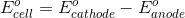Moreover, as was mentioned previously, since this is a voltaic cell the reaction will be spontaneous and thus thevalue must be positive.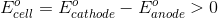Plugging in the value for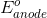gives us the following.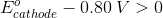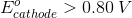This shows us that the cathode half-cell needs to have a compound with a standard reduction potential that is greater than silver's. The only answer choice that fits this criteria is cobalt, which has a standard reduction potential of.